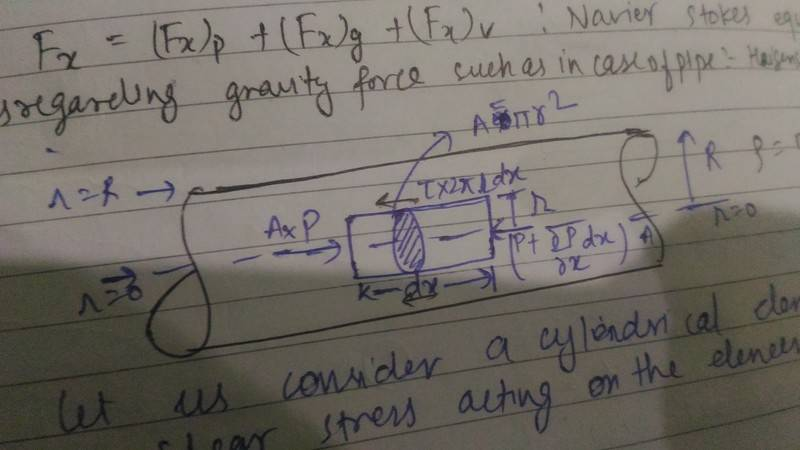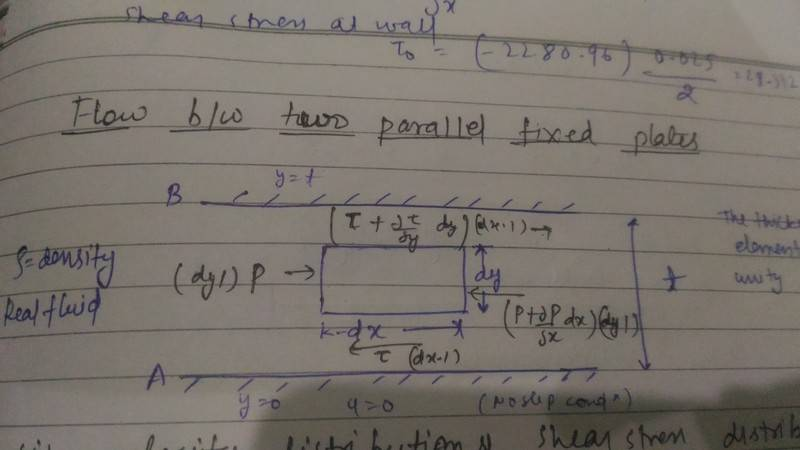# Shear stress direction and the velocity gradient

1)In a flowing fluid in laminar fashion we know that it flows in planes which slides over each other, Lets take a fluid element (cylindrical) in a pipe(Radius=R) the resistive force is (stress)(cross section area of cylinder with radius 'r') acting in backward direction, now if I take Flow between two parallel fixed plates and I take a element(cubical with thickness dy and depth (inside screen) to be unity we see that the direction of stress on the top and bottom face of element is opposite. How we are determining the direction of the stress?
2)lets take a fluid slab in a pipe (flowing fluid in it), the difference in velocity of top and bottom plane of slab is du and thickness between them is dy , then we know stress= (viscocity) (du/dy), what does this stress mean and where this stress is acting , on top of slab or bottom of slab , in forward or backward direction?#### Attachments

Chestermiller
Mentor
Are you asking about a radial shell of fluid situated between r and ##r+\delta r##, and are you asking about the shear stresses on the fluid within the shell at these inner and outer surfaces?

Umm no sir this is not the case , but I think understanding what you are telling can make me understand what Im asking.

Chestermiller
Mentor
Well, at ##r+\delta r##, the shear stress exerted by the fluid at larger radii on the fluid in the shell is given by:
$$\mu\frac{dv}{dr}\mathbf{i_z}$$where v is the axial velocity and ##\mathbf{i_z}## is a unit vector in the positive axial direction (flow direction). Since the fluid at radial locations greater than ##r+\delta r## is traveling slower than at radial location ##r+\delta r##, dv/dr is negative. This means that shear stress at this surface is in the negative axial direction. The fluid at greater radii are acting to slow down the fluid in the shell.

At radial location r (the inner surface of the shell), the shear stress exerted by the fluid at lesser radii on the fluid in the shell is given by:
$$\mu\frac{dv}{dr}(-\mathbf{i_z})$$. Since dv/dr is negative, this means that the shear stress at this surface is in the positive axial direction. The fluid at lesser radii are acting to speed up the fluid in the shell.

why the unit vector in second expression is negative?And the stress at r+dr, why we are only taking the effects of fluid which are greater than r+dr , cant the fluid inside also going to apply stress on it?

Chestermiller
Mentor
why the unit vector in second expression is negative?And the stress at r+dr, why we are only taking the effects of fluid which are greater than r+dr , cant the fluid inside also going to apply stress on it?
I'll answer your 2nd question first. We are doing a force balance on the fluid within the shell (like we did on rigid bodies in freshman physics). So we only include externally applied forces on the free body.

Your first question is a little more difficult to answer. It follows from the so-called Cauchy stress relationship which enables one to use the stress tensor to determine the stress on a surface of arbitrary orientation, exerted by the material on a specified side of the surface. Please google Cauchy stress relationship (aka Cauchy's Law), and see if you can follow what they are saying. I'll try to help if you have problems.

Last edited:
I tried to understand this relation , what I'm able to grasp is , It states that you don't have to find stress in all orientation(which can be in large number) , all you have to do is find the stress in the direction of the coordinate system and multiply it with unit normal vector (In matrix form), but sir how we gonna apply this concept in our problem

Chestermiller
Mentor
Have you learned about the stress tensor and how to represent a 2nd order tensor using dyadic notation? If not, please see Transport Phenomena by Bird, Stewart, and Lightfoot, Appendix A, section A.3 Tensor Operations in Terms of Components. After you are comfortable with that, I will show you how to determine the stress vector on a surface of arbitrary orientation, and then how to apply this to the specific problem at hand.

##\tau_{xy}##= This means the normal vector is in x direction and stress component is in y direction(If this is a cubical element face in x direction there will be ##\tau_{xx}## and ##\tau_{xz}## as well ). Is this what you asked? But still I will refer to the refrence .

Chestermiller
Mentor
##\tau_{xy}##= This means the normal vector is in x direction and stress component is in y direction(If this is a cubical element face in x direction there will be ##\tau_{xx}## and ##\tau_{xz}## as well ). Is this what you asked? But still I will refer to the refrence .
It's more general than that. Please be patient. What I'm going to show you is worth waiting for.

okay sir

This is really a great post you shared , It will take me a bit time to digest(I think I understood like 70%) . I need few hours to digest it completely , I will get back to you as soon as I get it . Thanks again

I have a doubt, sum of the traction vector=net force acting on the element?

Chestermiller
Mentor
I have a doubt, sum of the traction vector=net force acting on the element?
I don't quite understand your question.

I actually meant resultant of traction vector=net force acting on element?

Chestermiller
Mentor
I actually meant resultant of traction vector=net force acting on element?
I still don't understand. Can you state your doubt in the form of an equation?

like what I understood is ,you get stress vector of a plane(traction vector) by dotting the stress tensor with the normal vector . so I was thinking if we take resultant (like triangle law or parallelogram law) of all the traction vectors is this going to yield the net force per unit area on the element? .I also got that traction vector have 3 components in which two are shear stresses and one is normal stress .

Chestermiller
Mentor
Let's try this for your problem. The stress tensor for your laminar flow in a pipe system is given by: $$\boldsymbol{\sigma}=-p(\mathbf{i_r}\mathbf{i_r}+\mathbf{i_{\theta}}\mathbf{i_{\theta}}+\mathbf{i_z}\mathbf{i_z})+\tau_{rz}(\mathbf{i_r}\mathbf{i_z}+\mathbf{i_z}\mathbf{i_r})$$
OK so far?

Actually no , I don't get how this expression came. I can understand the terms that we took pressure in r,theta and z direction (normal and pressure are counter parallel to each other) , next term says shear stress in axial direction when normal is in radial and shear stress in radial direction when normal is in axial and the term inside bracket are dyadic they aren't operated as vectors multiplication(dot or cross). This is all I can get for now . Sorry this is literally very new for me ,maybe I'm gonna take more time than you expect from me.

Chestermiller
Mentor
No need to apologize. Let's continue assuming it is correct. What do you get when you dot this with ##\mathbf{i_r}## to determine the traction exerted on our shell at ##r+\delta r## by the fluid at radial locations ##>(r+\delta r)##?

##-pi_{r}## + ##\tau_{rz}i_{z}##

Chestermiller
Mentor
##-pi_{r}## + ##\tau_{rz}i_{z}##
Excellent. So this is the traction on our shell at ##r+\delta r## by the fluid external to the shell at larger radii. Note that since ##\tau_{rz}=\mu\frac{dv}{dr}## and since dv/dr is negative, the z-component of the traction vector is in the negative z direction.

Next, we would like to get the traction vector on the inner surface of the shell at r, exerted by the fluid at lesser radii. To get this traction vector, what would be the unit normal vector you use? What do you get when you dot the stress tensor at r with this unit normal vector?

I guess it will be ##-i_{r}## and this will give ##pi_{r}+\tau_{rz}(-i_{z})##

Chestermiller
Mentor
I guess it will be ##-i_{r}## and this will give ##pi_{r}+\tau_{rz}(-i_{z})##
Excellent again. So now I'm going to write the equilibrium force balance on your shell which is situated between r and ##r+\delta r## in the radial direction and between z and ##z+\delta z## in the axial direction:

$$[2\pi r \tau_{rz}]_{r+\delta r}\delta z\mathbf{i_z}+[2\pi r \tau_{rz}]_{r}\delta z(\mathbf{-i_z})+[(\pi(r+\delta r)^2-\pi r^2)p]_z\delta z\mathbf{i_z}+[(\pi(r+\delta r)^2-\pi r^2)p]_{z+\delta z}(-\mathbf{i_z})=\mathbf{0}$$

OK so far? Does this make sense to you?